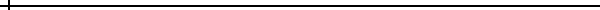Economics of Energy Efficiency

 This calculator determines the rate of return (interest earned) and the net present value of an energy-saving investment. Fill out the 'Inputs' section below and click the 'Calculate' button to complete the calculation.

Inputs

 Description You can enter a description of this calculation in the box below. Investment Cost of Measure Enter the up-front cost of the efficiency measure and the number of years that the measure will produce savings. Cost of Measure: \$ Life of Measure: years Annual Energy Savings Enter the dollars of energy savings that will be realized from the measure in the first year. Also, select how fast you think energy prices will increase in the future. First Year Savings: \$ Energy Price Inflation: 0% per year 1% per year 2% per year 3% per year 4% per year 5% per year 6% per year 7% per year 8% per year 9% per year 10% per year Annual Operating Cost Impact Enter any operating and maintenance cost impact caused by the efficiency measure (not counting energy savings). If operating and maintenance costs go up due to the measure, enter a positive number below. If operating costs decrease due to the measure, enter a negative number. Also, enter your estimate of how fast the impact will increase in future years due to price inflation. Operating Cost Impact: \$ Op. Cost Inflation: 0% per year 1% per year 2% per year 3% per year 4% per year 5% per year 6% per year Financing Interest Rate Enter the interest rate of the money that will be used to finance this efficiency measure. NOTE: This input is only used in the net present value calculation, not in the internal rate of return calculation. Financing Interest Rate: 2% per year 2.5% per year 3% per year 3.5% per year 4% per year 4.5% per year 5% per year 5.5% per year 6% per year 6.5% per year 7% per year 8% per year 9% per year 10% per year 12% per year 14% per year 16% per year 18% per year Click Here to Calculate the Results:Results

 Internal Rate of Return: This rate of return can be compared against the rates of returns or interest rates of alternative investments, such as stocks, bonds, certificates of deposit, etc. If energy costs are not tax deductible, which is the case with residential energy costs, the rate of return of the efficiency measure is an after-tax return. It should be compared against tax-free investments (e.g. tax-free municipal bonds) or against the after-tax rate of return of taxable investments. Net Present Value: \$ If the Net Present Value (NPV) is greater than \$0, then the energy efficiency measure provides a better return than the alternative investment. The NPV is calculated by adding together all the benefits of the efficiency measure over its life and subtracting out the costs. Benefits and costs are "discounted" to account for the time-value of money (the ability of money to earn interest). The Net Present Value is a better measure to use than Internal Rate of Return when comparing a number of possible efficiency measures that are "mutually exclusive", meaning only one can be implemented. For example, NPV is a good measure to use when trying to determine what level of insulation to add to an attic. Only one level of insulation can be chosen, i.e. they are mutually exclusive. Analyze each level of insulation as a separate calculation in the above calculator. Choose the level that produces the highest NPV.

If you have questions or comments, please send mail to alan@analysisnorth.com.